v0.3 (Feb 2022) Moved to R6 class system and implemented class grid, improved parameterisation of model.

v0.2 (Jan 2022) Added functionality to run single-period models.

v0.1 (Sep 2021) First release

# Introduction

There are a wide variety of sources of case data that are finely spatially-resolved and time stamped to monitor disease epidemiology in real-time. These data may include daily hospital admissions, positive tests, or calls to public health telephone services. Geospatial statistical models provide a principled basis for generating predictions of disease epidemiology and its spatial and temporal distribution from these data. rts2 provides a set of functions to conduct disease surveillance using a real-time feed of spatial or spatio-temporal data of cases.

The vignette describes spatio-temporal data analysis, however the package can also be used for single-period data with no time dimension. The analysis and data preparation is mostly the same, but any exception are noted in the text below.

## Installation and dependencies

The two main dependencies are sf and rstan (or cmdstanr).

### sf

sf can be installed as normal:

install.packages("sf")

### rstan

The rts2 package uses Stan, which is a probabilistic programming language used to draw posterior samples from Bayesian models. rstan is an R package to interface with Stan. The trickiest part of the installation is getting a C++ compiler working with R. The easiest way of doing this on Windows is to install Rtools - see https://github.com/stan-dev/rstan/wiki/RStan-Getting-Started for a detailed description. Then, the rstan package can be installed as normal:

install.packages("rstan")

### cmdstanr

The package can be used with cmdstanr instead of rstan. cmdstanr is not necessary to run the package so if you’ve gotten rstan working then you could skip this section. cmdstanr is lightweight, it can compile models much faster than rstan, and gets updates sooner, so it is worth looking into. Installing cmdstan and cmdstanr is straightforward:

install.packages("cmdstanr", repos = c("https://mc-stan.org/r-packages/", getOption("repos")))

and then to install cmdstan:

cmdstanr::install_cmdstan()

### rts2

When all these packages are installed, rts2 can be installed (the package will be submitted to CRAN very soon). The simplest method on a Windows machine is to download the binary at this link and then install as (changing the file path to the location of the downloaded file)

install.packages("./rts2_0.3.zip",repos=NULL)

Alternatively you can compile from source using:

devtools::install_github("samuel-watson/rts2")

# R6 grid class

The basis of this package is the class grid. An object of this class holds the geographic data as an sf object and has several functions that modify the data to add on the case counts and covariate data. The user can then call lgcp_fit() directly on the object to generate a model fit. The class also contains several analysis and results generating functions.

# Data preparation

For the analysis, we will need an sf object with our grid geography, case counts, and any covariates. The package provides some simple functions for this purpose. To start we will need:

1. The boundary of the area of interest
2. Geocoded case data
3. Covariate data

## Boundary

If you don’t have a boundary there are different ways of creating them.

In the UK, the Ordinance Survey provide a comprehensive set of boundary data here: https://www.ordnancesurvey.co.uk/business-government/products/boundaryline. The st_read() function will read in shapefiles to sf format.

For non-standard geographies, i.e. areas that are not standard political entities, such as the catchment area of a hospital or sections of a city, one will need to create the boundary. Shape files are relatively straightforward to generate by hand using GIS software such as QGIS or ArcGIS. Typically the user can load Open Street Map or aerial imagery data into the GIS software, create a new polygon layer, and then export this to an ESRI shapefile that can be read by R. Some resources for doing this with OpenStreetMap data can be found at https://wiki.openstreetmap.org/wiki/Shapefiles#Create_your_own_shapefiles. User generated geographies may be necessary for low income settings and emergency response settings such as refugee camps.

### Combining smaller areas

In some cases we may have the geography of lots of smaller areas that we can combine into the area of interest. For example, for England and Wales one can identify the LSOAs that are in the area of interest and extract the boundary information. (You can download the LSOAs and related covariates from: http://www.sam-watson.xyz/lsoa). We can then subset the LSOAs in our area of interest and take their union to create a boundary. For example, to create a boundary for the city of Birmignham

grid_data$plot()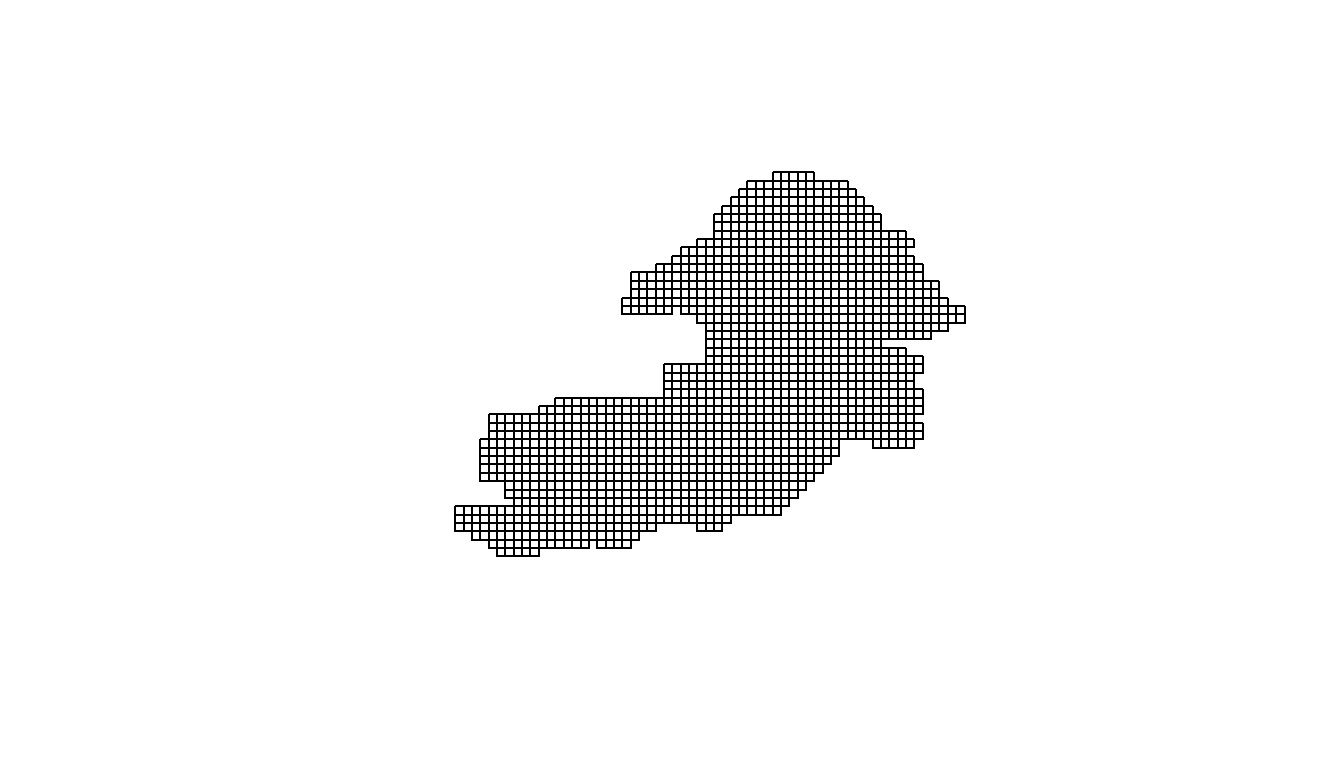Note that we can use other plotting tools to visualise this grid over a map if needed, see the Visualisation section below. ## Overlaying the points onto the grid Next we have to generate counts of the numbers of cases in each grid cell at each time point. First, we create a spatial object from our case data: point_data <- create_points(df, pos_vars = c("lat","long"), t_var = c("admit")) ## Using lat as y-coordinate and long as x-coordinate. plot(point_data)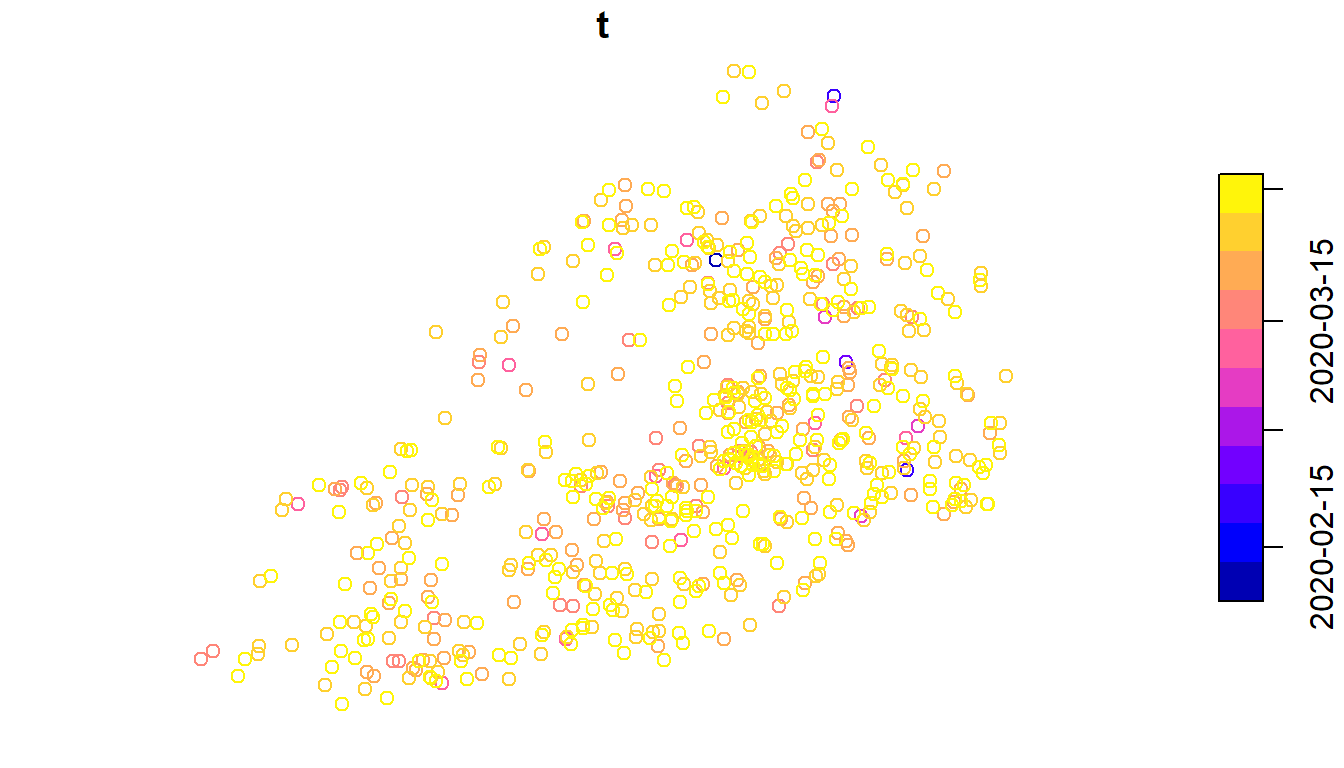Note that if we are conducting a spatial only analysis, then t_var should be NULL. Now we have our points we can add them into our grid. For spatio-temporal analysis, we can determine here what size time step we want (day, week, or month) and how many previous periods to include (laglength). The choice of lag length is similar to the choice of cell size, we want it to be long enough to provide the best predictions of current incidence, but as short as possible other wise to minimise the required compuational resources. We found that about 14 days of lag provided good predictions. Note that the computational time is linear in the number of time periods so the costs of going too high are not too bad! To map the points onto our grid and add columns for each time period: grid_data$points_to_grid(point_data,
t_win = "day",
laglength = 14)
## Warning in grid_data$points_to_grid(point_data, t_win = "day", laglength = 14): ## CRS not equal. Setting st_crs(point_data)==st_crs(self$grid_data)
## added points data to grid data

The arguments t_win and laglength can be ignored for spatial-only analyses.

The output of this function is identical to grid_data but with additional columns (labelled t* for spatio-temporal or y for spatial-only). You can see that the grid data now contains a column for each time period with the count in each cell as well as the date for that column.

colnames(grid_data$grid_data) ##  "bgrid" "t1" "date1" "t2" "date2" "t3" "date3" "t4" ##  "date4" "t5" "date5" "t6" "date6" "t7" "date7" "t8" ##  "date8" "t9" "date9" "t10" "date10" "t11" "date11" "t12" ##  "date12" "t13" "date13" "t14" "date14" The highest number is the most recent period, so to visualise the counts for April 1st 2020: grid_data$plot("t14")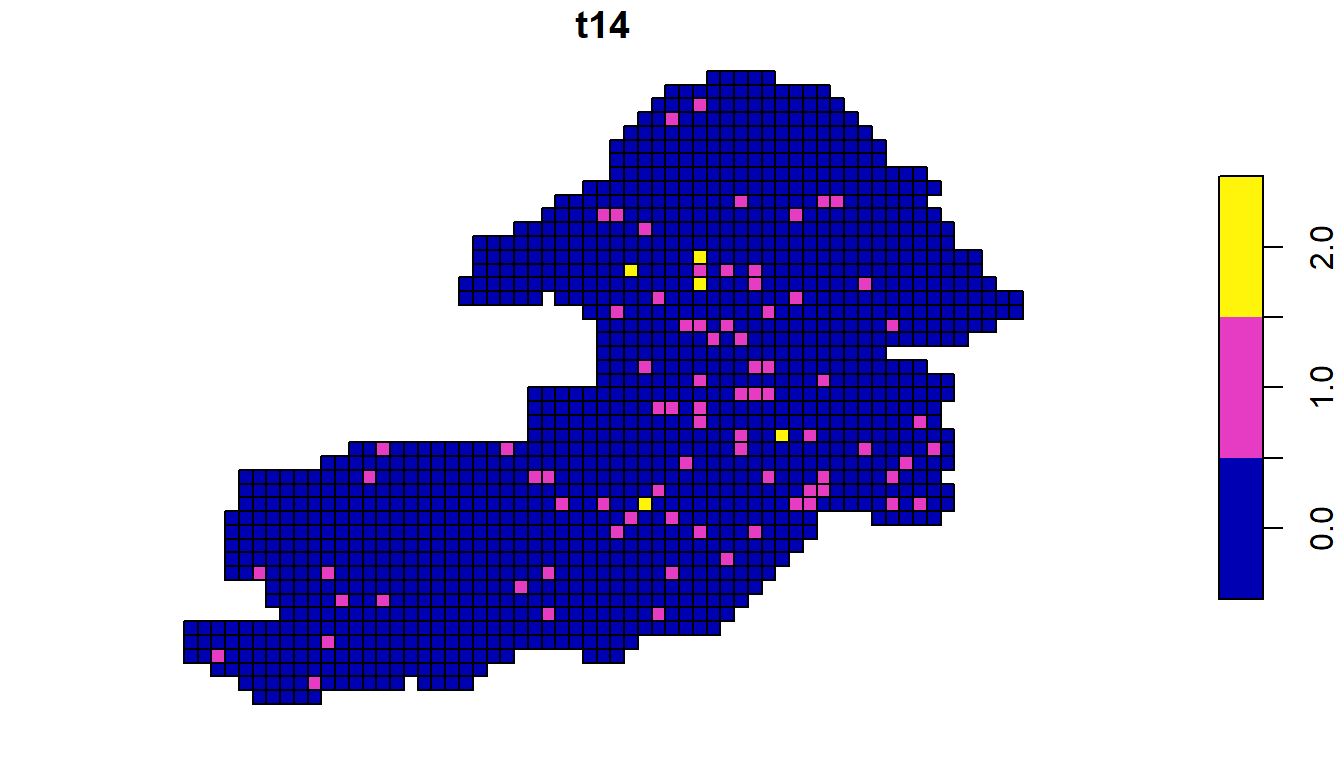## Overlaying covariate data

We can include covariates in our model. The aim of including covariates is not to provide inference about the effect of any particular covariate but to improve predictions. For example, by including day of the week as a covariate we can capture differences in hospital attendances and admissions resulting from individual behaviour not related to variations in disease incidence. At a minimum we would suggest including population density to enable population standardised predictions. LSOA data for the whole UK can be downloaded as a SpatialPolygonsDataFrame from http://www.sam-watson.xyz/lsoa. The LSOA file can be read in an converted to the appropriate type as:

lsoa <- load(gzfile(LSOA_FILE_PATH))
lsoa <- st_as_sf(lsoa)

Note that this is a very large file so we may want to cut it down to size with out boundary:

idx <- st_intersects(y=lsoa,x=boundary)
idx <- unlist( sapply( idx, [) )
lsoa <- lsoa[idx,]

Covariates can be added to the grid data using the function add_covariates(). There are three main types of covariate.

### Spatially-varying only covariates

Where the time step is short, such as days, it is unlikely there will be data that varies by time step over the area of interest. For example, population density data will be static over the area of interest. To add spatially-varying covariates to the grid data we require an sf object with polygons encoding the covariate data. The add_covariates() function then, for each grid cell, takes an average of the values in the overlapping polygons, weighted by either the size of the area of overlap or the population, if population density data are available. For the UK, we can use the same LSOA data as before. Another good source of population density predictions is WorldPop, which will provide rasters at 100m or 1km resolution for all countries https://www.worldpop.org/.

First, we add the population density:

grid_data$add_covariates(lsoa, zcols="popdenshect", weight_type="area", verbose=FALSE) where lsoa' is the LSOA data,zcols’ specifies the name(s) of the column(s) of the covariates we want to add, and type indicates that we want to average with respect to the area. The original LSOA data and its projection on the grid look like this: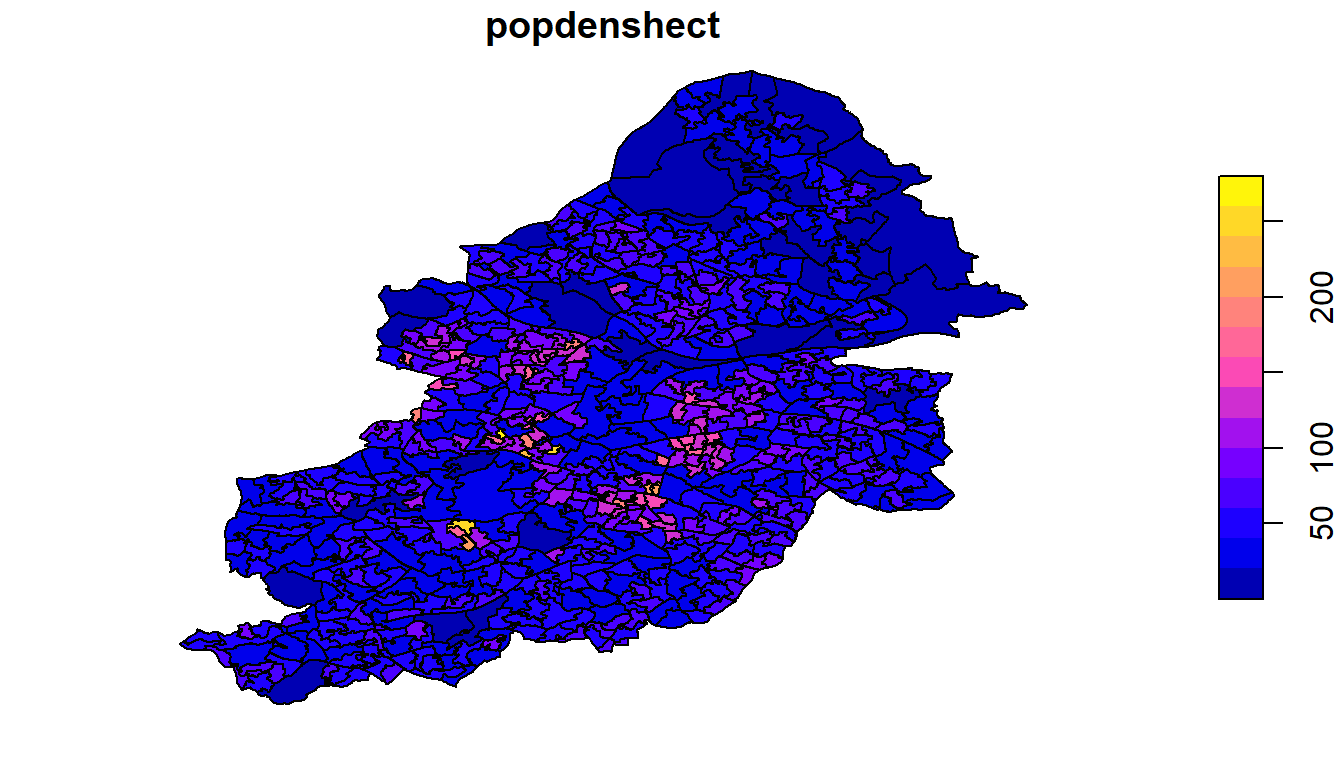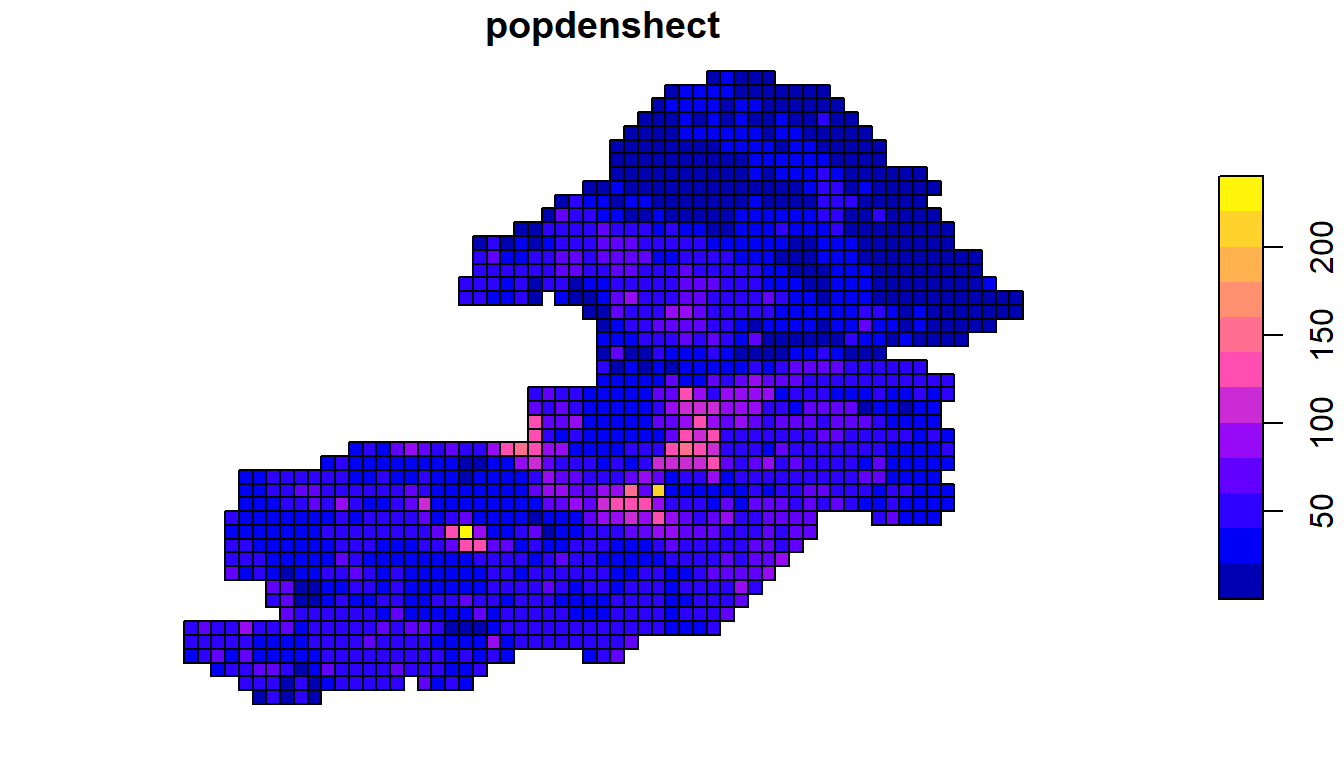We will next add a covariate that shows the proportion of the population aged over 65 prop65. We want this covariate to be a population-weighted rather than area-weighted average as it is a feature of the population: grid_data$add_covariates(lsoa,
zcols="prop65",
weight_type="pop",
popdens = "popdenshect",
verbose = FALSE)

which produces:

grid_data$plot("prop65")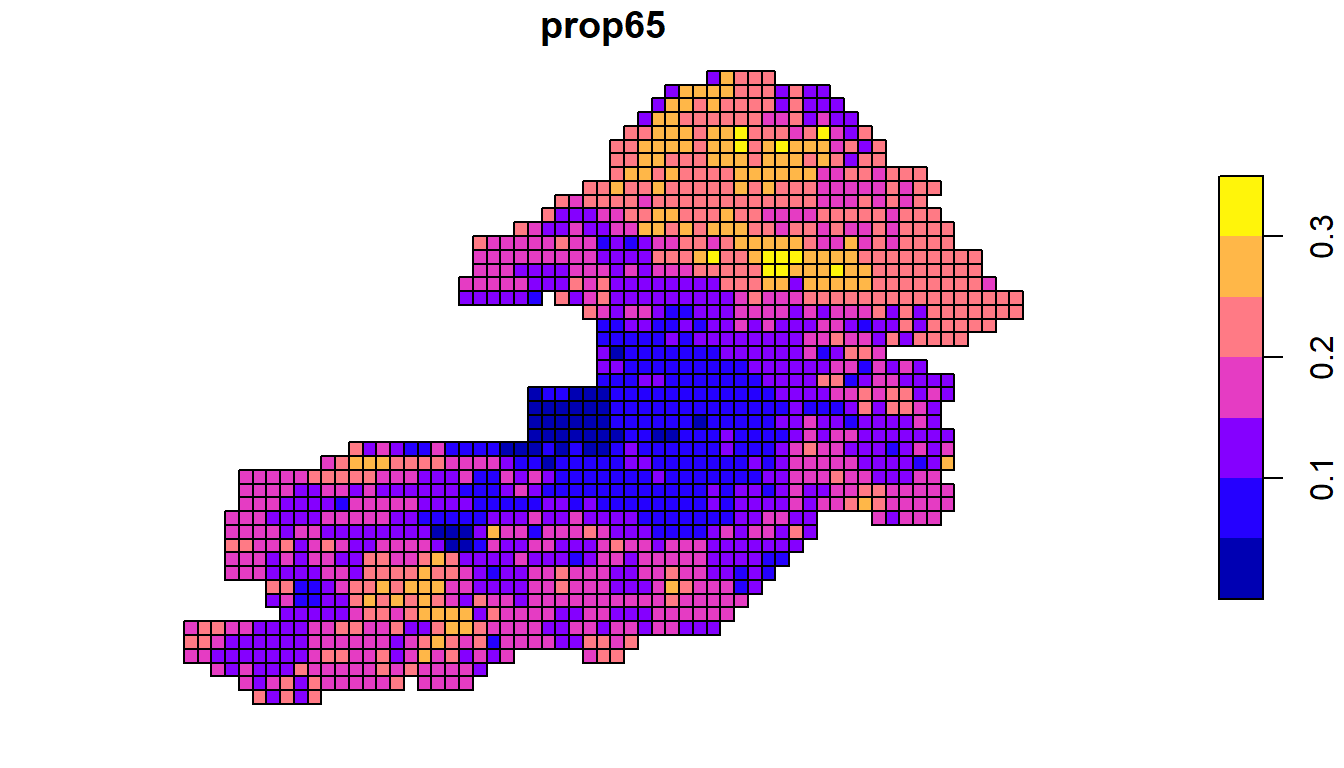### Temporally-varying only covariates Some covariates, like day of the week, vary over time but are the same across the area for all. To add a temporally-varying covariate we need to produce a data frame with a column for time period (from 1 to laglength) and then columns with the values of the covariates. For day of the week we can use the package function: df_dow <- grid_data$get_dow()
print(df_dow)
##     t day dayFri dayMon daySat daySun dayThu dayTue dayWed
## 1   1 Thu      0      0      0      0      1      0      0
## 2   2 Fri      1      0      0      0      0      0      0
## 3   3 Sat      0      0      1      0      0      0      0
## 4   4 Sun      0      0      0      1      0      0      0
## 5   5 Mon      0      1      0      0      0      0      0
## 6   6 Tue      0      0      0      0      0      1      0
## 7   7 Wed      0      0      0      0      0      0      1
## 8   8 Thu      0      0      0      0      1      0      0
## 9   9 Fri      1      0      0      0      0      0      0
## 10 10 Sat      0      0      1      0      0      0      0
## 11 11 Sun      0      0      0      1      0      0      0
## 12 12 Mon      0      1      0      0      0      0      0
## 13 13 Tue      0      0      0      0      0      1      0
## 14 14 Wed      0      0      0      0      0      0      1

Then to add these columns to our main grid data:

grid_data$add_covariates(df_dow, zcols=c("dayMon","dayTue", "dayWed","dayThu","dayFri", "daySat","daySun")) ## added covariates dayMonadded covariates dayTueadded covariates dayWedadded covariates dayThuadded covariates dayFriadded covariates daySatadded covariates daySun ### Spatially and temporally varying covariates In some cases we may have covariates that vary over time and space. There are two ways of adding these into the main grid data. Both ways need to generate for each covariate as many columns as there are time periods, which have the variable name followed by the time period index in the same way that the temporally-varying covariates are included: colnames(grid_data$grid_data)
##    "bgrid"       "t1"          "date1"       "t2"          "date2"
##    "t3"          "date3"       "t4"          "date4"       "t5"
##   "date5"       "t6"          "date6"       "t7"          "date7"
##   "t8"          "date8"       "t9"          "date9"       "t10"
##   "date10"      "t11"         "date11"      "t12"         "date12"
##   "t13"         "date13"      "t14"         "date14"      "popdenshect"
##   "prop65"      "dayMon1"     "dayMon2"     "dayMon3"     "dayMon4"
##   "dayMon5"     "dayMon6"     "dayMon7"     "dayMon8"     "dayMon9"
##   "dayMon10"    "dayMon11"    "dayMon12"    "dayMon13"    "dayMon14"
##   "dayTue1"     "dayTue2"     "dayTue3"     "dayTue4"     "dayTue5"
##   "dayTue6"     "dayTue7"     "dayTue8"     "dayTue9"     "dayTue10"
##   "dayTue11"    "dayTue12"    "dayTue13"    "dayTue14"    "dayWed1"
##   "dayWed2"     "dayWed3"     "dayWed4"     "dayWed5"     "dayWed6"
##   "dayWed7"     "dayWed8"     "dayWed9"     "dayWed10"    "dayWed11"
##   "dayWed12"    "dayWed13"    "dayWed14"    "dayThu1"     "dayThu2"
##   "dayThu3"     "dayThu4"     "dayThu5"     "dayThu6"     "dayThu7"
##   "dayThu8"     "dayThu9"     "dayThu10"    "dayThu11"    "dayThu12"
##   "dayThu13"    "dayThu14"    "dayFri1"     "dayFri2"     "dayFri3"
##   "dayFri4"     "dayFri5"     "dayFri6"     "dayFri7"     "dayFri8"
##   "dayFri9"     "dayFri10"    "dayFri11"    "dayFri12"    "dayFri13"
##  "dayFri14"    "daySat1"     "daySat2"     "daySat3"     "daySat4"
##  "daySat5"     "daySat6"     "daySat7"     "daySat8"     "daySat9"
##  "daySat10"    "daySat11"    "daySat12"    "daySat13"    "daySat14"
##  "daySun1"     "daySun2"     "daySun3"     "daySun4"     "daySun5"
##  "daySun6"     "daySun7"     "daySun8"     "daySun9"     "daySun10"
##  "daySun11"    "daySun12"    "daySun13"    "daySun14"

If the covariate data are included in different data frames for each time period, then we can repeat the process above for each time period and add a label using the t_label argument:

grid_data$add_covariates(lsoa_t1, zcols=c("covA","covB",...), t_label = 1) Alternatively, if the value of the covariates are all included in different columns of the same data frame then we can add them in one go, ensuring we keep to the same naming convention: grid_data$add_covariates(lsoa_t1,
zcols=c("covA1","covA2","covA3",...))

# Running the model

Now grid_data contains everything we need to run the model. The function we need is lgcp_fit. We will need to tell it what the population density variable is and what the covariates are to include. In addition there are three other key arguments. The statistical model used for analysis involves an approximation to the complete model to dramatically improve running time. The approximation involves using a linear sum of “basis functions”. The first two parameters control this sum (see Statistical Model section below, as well as references  and  for more information):

• m: The number of basis functions. The higher the number of basis functions, the more accurate, but the slower the approximation runs. If $$n$$ is the number of grid cells, $$T$$ the number of time periods, then the computational time increases approximately as $$O(nTm^2 + m^2)$$. There is no easy answer to the best number to include here as it depends on the “wiggliness” of the surface we are trying to model. If we suspect that the risk varies very smoothly across our area of interest then a small $$m$$ will suffice. Reference  shows some simulations with different values of $$m$$. We have found between 10 and 15 to work well.

• L: The proportionate extension of the boundary. The approximation models the locations of the grid cells with respect to the edges of a square around the area. If the edges of this square are at the edge of the area of interest then we can get poor predictions near the edge. We therefore want to extend the square. For the analysis the coordinates are scaled to $$[-1,1]$$ in each dimension, the square is set to have dimension $$[-L,L]$$. As before, reference  shows some simulations with different values of $$L$$. We have found between 2 and 3 to work well.

We can also specify prior distributions for our model parameters, if these are not set then the default priors described in the Statistical Model section will be used. The priors should be provided as a list:

grid_data$priors <- list( prior_lscale=c(0,0.5), prior_var=c(0,0.5), prior_linpred_mean=c(-5,rep(0,7)), prior_linpred_sd=c(3,rep(1,7)) ) where these refer to the priors: • prior_lscale: the length scale parameter has a half-normal prior $$l \sim N(a,b^2)I[0,\infty)$$. The vector is c(a,b). • prior_var: the standard deviation term has a half normal prior $$\sigma \sim N(a,b^2)I[0,\infty)$$. The vector is c(a,b). *prior_linpred_mean and prior_linpred_sd: The parameters of the linear predictor. If $$X$$ is the $$nT \times Q$$ matrix of covariates, with the first column as ones for the intercept, then the linear prediction contains the term $$X'\gamma$$. Each parameter in $$\gamma$$ has prior $$\gamma_q \sim N(a_q,b_q^2)$$. prior_linpred_mean should be the vector $$(a_1,a_2,...,a_Q)$$ and prior_linpred_sd should be $$(b_1,b_2,...,b_Q)$$. On the initial run the priors will need to be set. But in subsequent analyses the posteriors from previous runs can be used as priors. The function extract_priors() will take a model run and generate a list in the same format as above ready for use in the next run. For example, grid_data$priors <- extract_priors(res)

## Call to Stan

The call to run the model is then

res <- grid_data$lgcp_fit(grid_data, popdens="popdenshect", covs=c("dayMon","dayTue","dayWed", "dayThu","dayFri","daySat", "prop65"), m = 10, L = 2) where we have also told it what the name of the variable for population density is, and the list of the names of the covariates. For temporally-varying covariates we only need to include the stem of the name rather than all columns numbered by time period, for example, only dayMon rather than dayMon1, dayMon2 etc. The function processes the data and runs the Stan program. The first time you run the model, it may re-compile the program. One can control the sampling parameters (number of chains, iterations etc.) using the same arguments for cmdstanr, see help($sample) for more details. In comparison to the sampler from the previous realTimeSurv package, Stan requires far fewer iterations to achieve an equivalent effective sample size owing to its implementation of Hamiltonian Monte Carlo and other methods.

## Running time

Running time can vary a lot. As well as the number of observations and parameters of the approximation, it can also depend on how well specified the model is. As described above though, the model running time should scale approximately linearly with the number of observations and time periods. To give an indication of running time, the example model, with 2,510 grid cells, 14 time periods, and 10 basis functions, with 500 warmup and sampling iterations, took around 2 to 4 hours to run to achieve good convergence and generate a reasonable number of posterior samples. This compares to 8 to 10 hours required by the sampler in the realTimeSurv and lgcp packages.

## Saving the output

The output of the sampling function is a stanfit or cmdstanr environment, depending on the sampler used (see help(lgcp_fit)). If using cmdstanr and the dir argument is unspecified then the output will be lost when the session is ended. We recommend saving the output so it can be recalled in other sessions, see https://mc-stan.org/cmdstanr/reference/fit-method-save_output_files.html for information how to save and read these model outputs.

# Analysing the output

## Predictions

The output from the model enables us to generate four main predictions across our area of interest:

• Predicted total incidence.
• Predicted incidence per head of population.
• The relative risk, i.e. the relative difference between predicted and expected incidence. For example, a relative risk of 2 would imply that the predicted incidence is twice a high as would be expected given the area mean and local covariate values.
• The incidence rate ratio relative to some previous time point for spatio-temporal analyses. For example, we may be interested in comparing incidence to seven days prior; a value of 1.5 would imply that the incidence has risen 50% in a week.

We can extract any or all of these predictions using the function extract_preds(). The first time it runs it may take a few minutes as the posterior draws are read into R:

grid_data$extract_preds(stan_fit = res, type = c("pred","rr","irr"), irr.lag = 7, popdens = "popdenshect") Where we provide the original grid data, the stan fit, and the outputs we want (type). The function will output the columns, which map onto the list above: * pred_mean_total and pred_mean_total_sd * pred_mean_pp and pred_mean_pp_sd * rr and rr_sd * irr and irr_sd ## Hotspots The concept of a “hotspot” is often poorly or vaguely defined and it can mean different things to different people. Generally, we can define it as an area where there is a high probability that some epidemiological measure(s) exceed predefined threshold(s). Here, we can use any of the outputs of the model listed above (incidence, relative risk, or incidence rate ratio). The hotspot() function allows us to define a hotspot based on any combination of these criteria (connected logically by “and”). For example, grid_data$hotspots(stan_fit=res,
irr.threshold = 1.5,
irr.lag = 7,
col_label="high_irr")

will return the probability that the IRR relative to seven days prior is greater than 1.5 and call the column high_irr. The call

grid_data$hotspots(stan_fit=res, irr.threshold = 1.5, irr.lag = 7, rr.threshold = 2, col_label="high_irr_and_rr") will return the probability that the IRR relative to seven days prior is greater than 1.5 and that the relative risk is greater than 2 and label the column high_irr_and_rr. ## Plotting and visualisation As the output of the analysis is an sf object, there are many different tools we can use to plot and visualise the results. ### Default plot We can use the default plot function, for example, here for population standardised incidence: grid_data$plot("pred_mean_pp")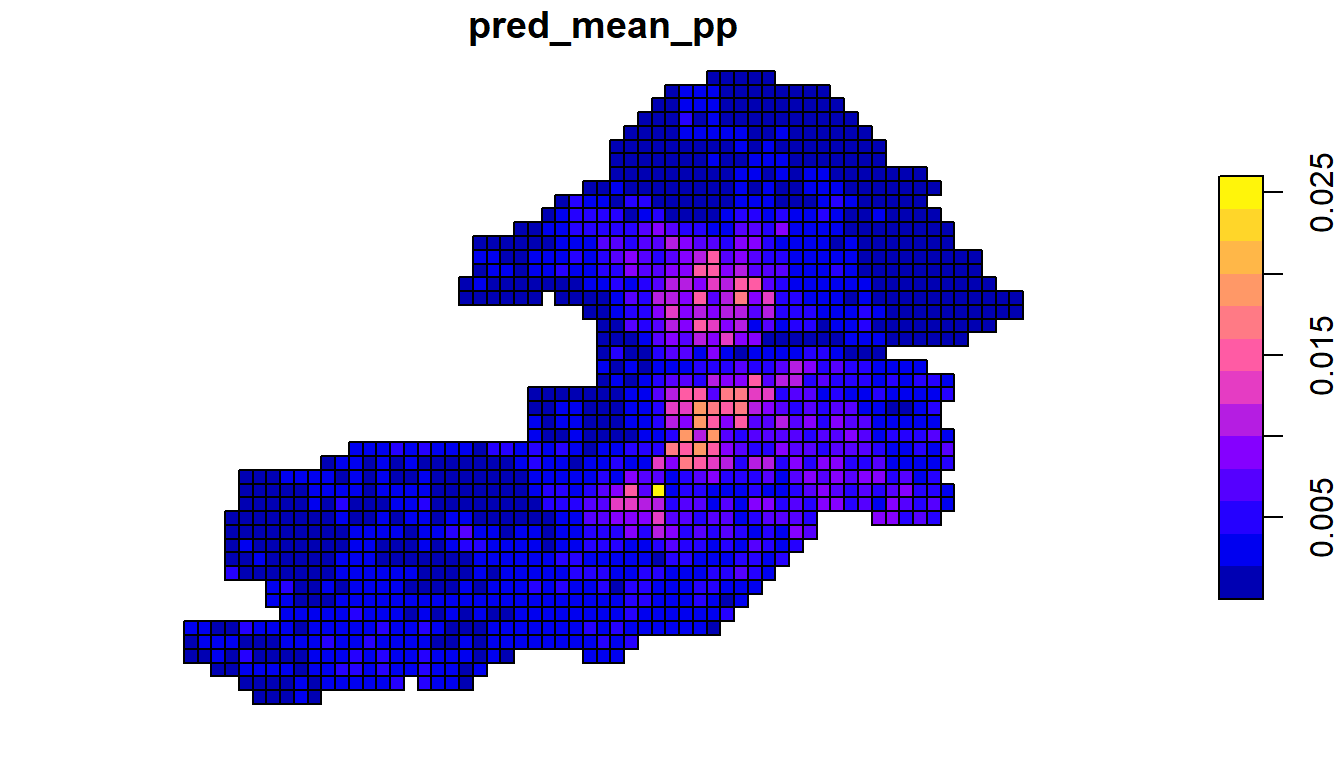### tmap

The package tmap provides a range of tools for map plotting including allowing multiple panels to be plotted. Here, we’ll visualise our two hotspots. For this application we will consider that areas where the probability exceeds 80% that the IRR or RR is greater than our thresholds is a hotspot. We’ll use a colour pallette from the colorRamps package that highlights these areas. Then we’ll use the tmap plotting functions where we’ve expanded the bounding box to fit in the legend:

require(colorRamps)
## Loading required package: colorRamps
pal <- c(blue2red(16)[1:8],blue2red(5)[4:5])

require(tmap)
## Loading required package: tmap
## Warning: package 'tmap' was built under R version 4.1.2
stbr <- st_bbox(grid_data$grid_data) stbr <- stbr+ (stbr-stbr)*0.5 tm_shape(grid_data$grid_data,
bbox=stbr)+
tm_polygons(c("high_irr",
"high_irr_and_rr"),
palette=pal,
breaks=seq(0,1,0.1))+
tm_layout(legend.position = c("right", "bottom"))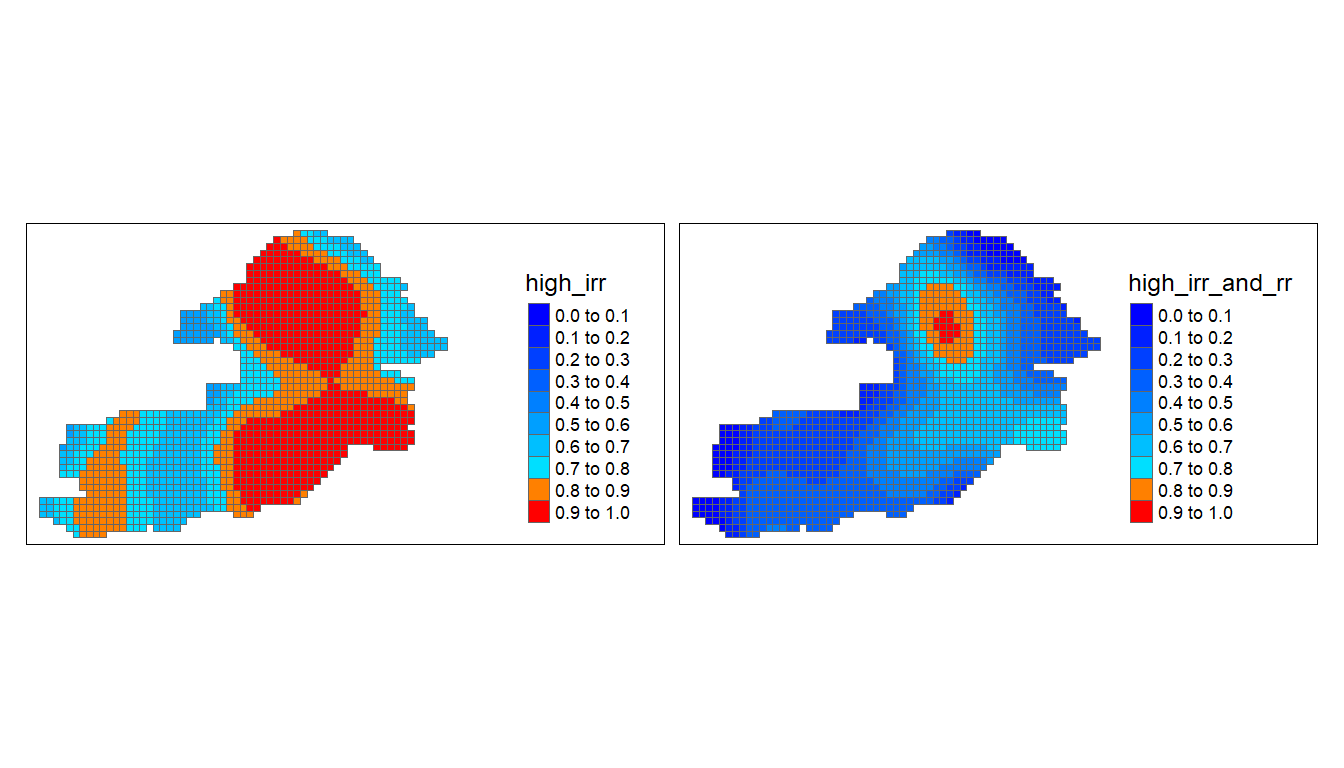### mapview

One can create interactive overlays of the data on OpenStreetMap maps using the mapview package. For example,

require(mapview)
map1 <- mapview(grid_data\$grid_data,
zcol=c("high_irr_and_rr"),
map.types="OpenStreetMap",
col.regions=pal,
layer.name="Hotspot",
label="name")
mapshot(map1)

A screenshot of the model output is shown below.